Neural Networks and Deep Learning 是由 Michael Nielsen 编写的开源书籍，这本书主要讲的是如何掌握神经网络的核心概念，包括现代技术的深度学习，为你将来使⽤神经网络和深度学习打下基础，以下是我的读书笔记。

• 人类视觉系统：通过数十亿年不断地进化与学习，最终能够极好地适应理解视觉世界的任务，从而无意识地就可以对目标进行判断识别
• 神经网络：通过提供的样本来推断出识别某种目标的规则，作为判断标准

• 两个重要的人工神经元：感知器和S型神经元
• 神经⽹络的架构
• ⼀个简单的分类⼿写数字的⽹络
• 标准的神经网络学习算法：随机梯度下降算法

## 感知器

1943年，心理学家McCulloch和数学家Pitts发表了《A logical calculus of the ideas immanent in nervous activity》，其中提出了抽象的神经元模型MP，但是在这个模型中权重都是要求提前设置好才可以输出目标值，所以很遗憾，它不可以学习，但这不影响此模型给后来者带来的影响，比如感知器：$x_{1},x_{2},x_{3}$ 分别表示三个不同的二进制输入，output则是一个二进制输出，对于多种输入，可能有的输入成立有的不成立，在这么多输入的影响下，该如何判断输出output呢？Rosenblatt引入了权重来表示相应输入的重要性。• $x=(x_{1},x_{2},…,x_{j})$
• $w=(w_{1},w_{2},…,w_{j})$$x$ $y$ $output$
0 0 0
0 1 1
1 0 1
1 1 0

## S型神经元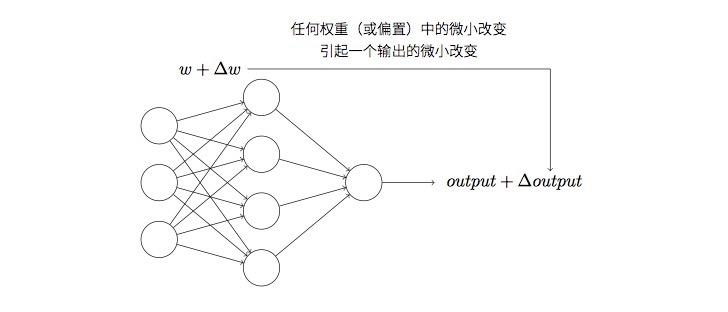$$\sigma(z) \equiv \frac{1}{1+e^{-z}}$$

$$z=\sum_{j} w{_j}x{_j}+b$$

$$\frac{1}{1+\exp(-\sum_j w_j x_j-b)}$$

S型神经元的输出是[0,1]之间的任何实数，那么这个分类模型该如何判断分类结果呢？其实很简单，我们可以定义一个数值，比如：

• $\sigma(z) \leq 0.5$ 输出0
• $\sigma(z)>0.5$ 输出1

## 神经⽹络的架构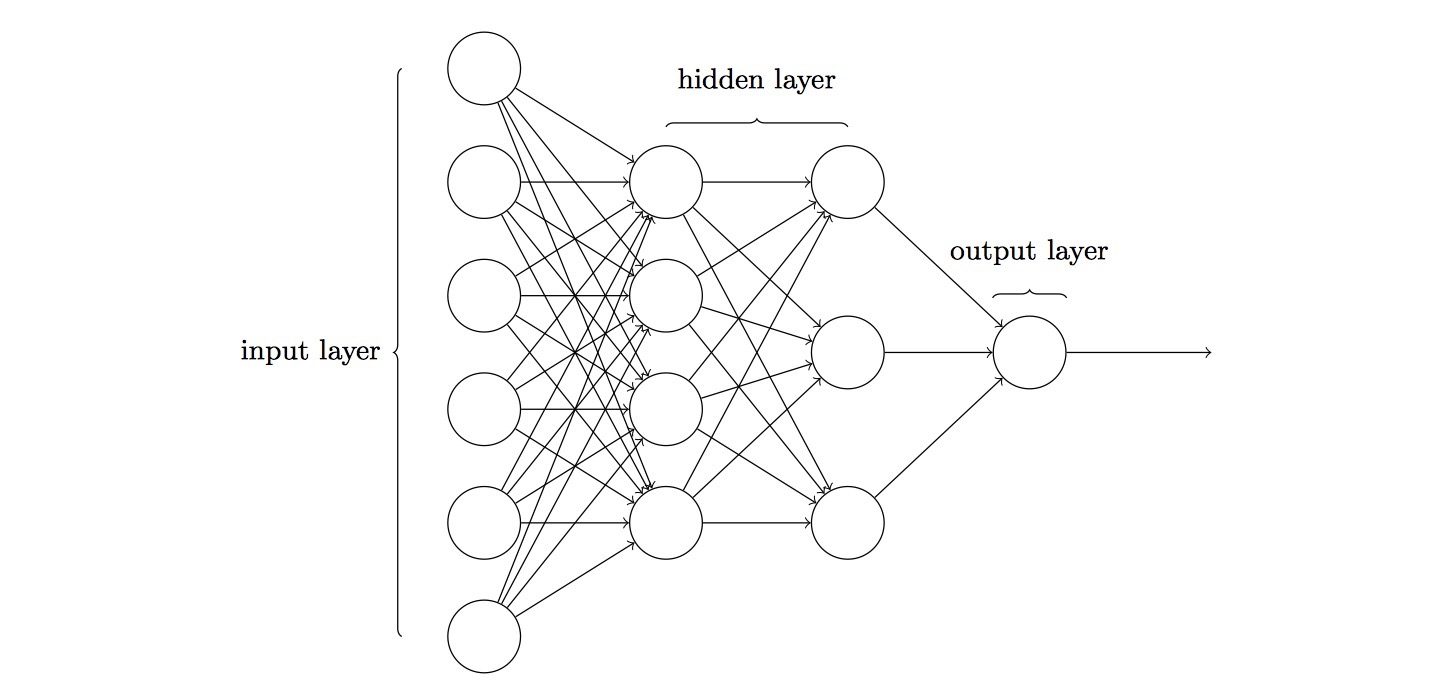• ⽹络中最左边的称为输⼊层，其中的神经元称为输⼊神经元
• 中间得称之为隐藏层，图中有两个隐藏层
• 最右边称之为输出层
• 上⼀层的输出作为下⼀层的输⼊，信息总是向前传播，从不反向回馈：前馈神经网络
• 有回路，其中反馈环路是可⾏的：递归神经网络

## ⼀个简单的分类⼿写数字的⽹络

• 504192是连续在一起的图像，首先可以将其分割成6个小图像，比如5 0 …等

• 再对分割开的小图像进行分类，比如识别5我们将使⽤⼀个三层神经⽹络来识别单个数字：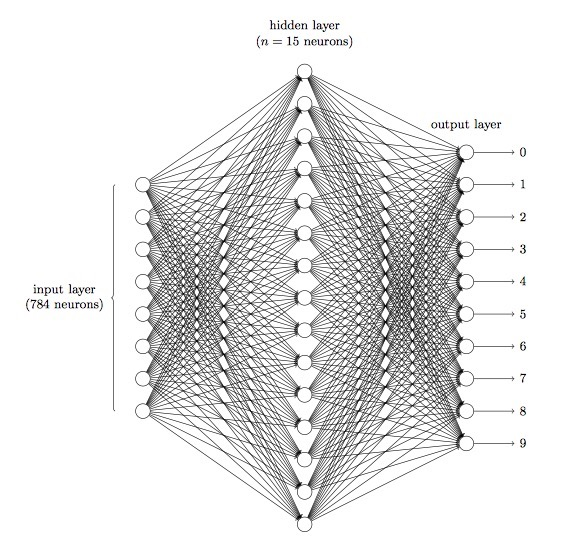由于训练数据由$28*28$的⼿写数字的图像组成，所以:

• 输入层有784个神经元，因为$784 = 28 \times 28$，输⼊像素是灰度级的，值为0:0表⽰⽩⾊，值为1:0表⽰⿊⾊，中间数值表⽰逐渐暗淡的灰⾊

• 隐藏层⽤n来表⽰神经元的数量，我们将给n实验不同的数值。⽰例中⽤⼀个⼩的隐藏层来说明，仅仅包含$n = 15$个神经元

• ⽹络的输出层包含有10个神经元，如果第一个输出神经元被激活，那么数字就是0，以此类推，从0到9

## 随机梯度下降算法

 1 2 3 4 5 6 7  pylab └── datasets └── MNIST_data ├── t10k-images-idx3-ubyte.gz ├── t10k-labels-idx1-ubyte.gz ├── train-images-idx3-ubyte.gz └── train-labels-idx1-ubyte.gz 

 1 2 3 4 5  from matplotlib import pyplot as plt from tensorflow.examples.tutorials.mnist.input_data import read_data_sets mnist = read_data_sets("../datasets/MNIST_data/", one_hot=True) 

  1 2 3 4 5 6 7 8 9 10 11 12 13 14 15 16 17 18 19 20 21 22  print("Training data size: %s" % mnist.train.num_examples) print("Validating data size: %s" % mnist.validation.num_examples) print("Testing data size: %s" % mnist.test.num_examples) # 每张图片是长度为784的一维数组 print(len(mnist.train.images)) def display_digit(image): image = image.reshape([28,28]) plt.imshow(image, cmap=plt.get_cmap('gray_r')) plt.show() print("样本真实数字为：%s" % list(mnist.train.labels).index(1)) # 显示图像 display_digit(mnist.train.images) # Output # Training data size: 55000 # Validating data size: 5000 # Testing data size: 10000 # 784 # 样本真实数字为：3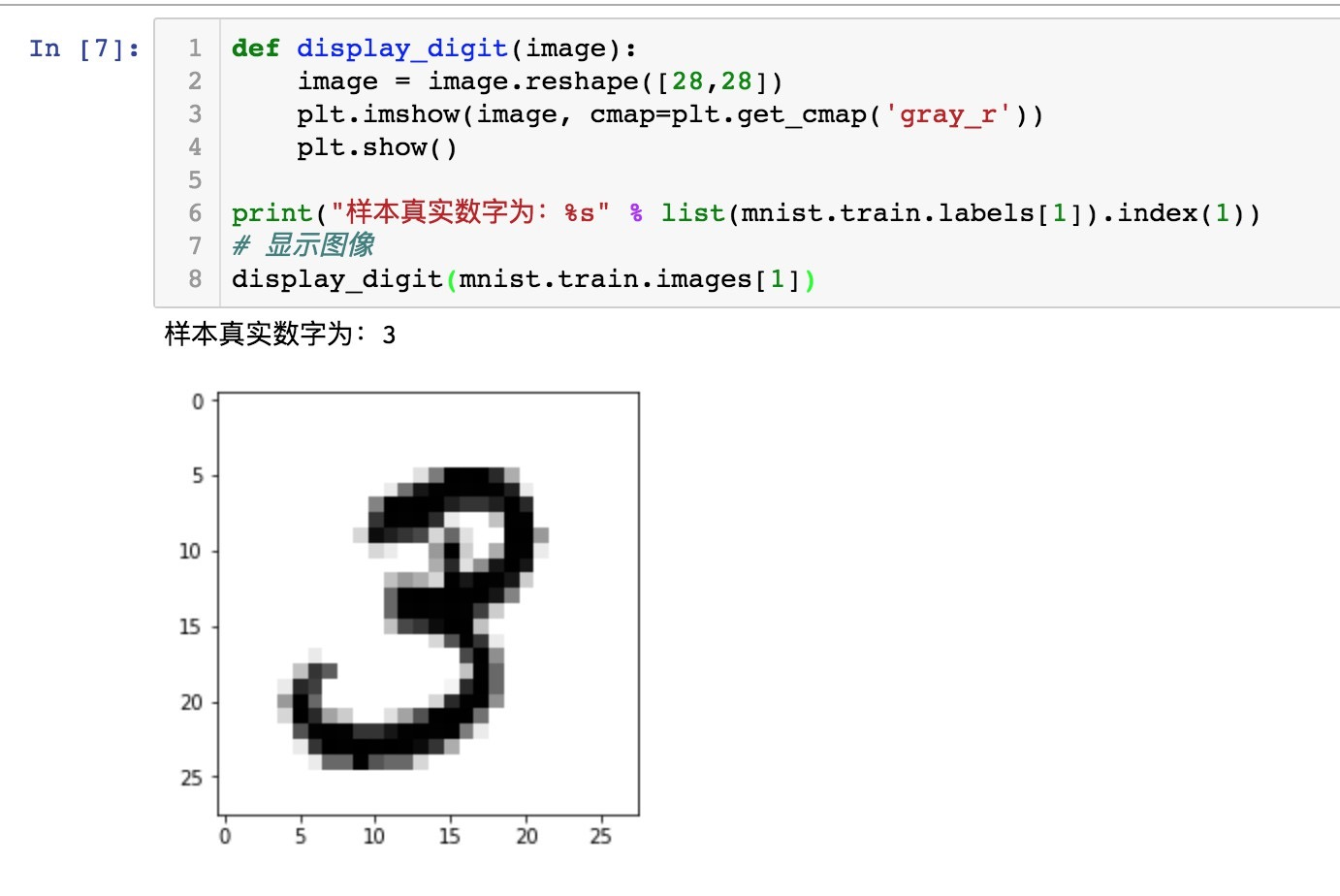$$C(w,b) \equiv \frac{1}{2n} \sum_x | y(x) - a|^2$$

• w 表⽰所有的⽹络中权重的集合
• b 是所有的偏置
• n 是训练输⼊数据的个数
• a 是表⽰当输⼊为x时输出的向量，可以理解为output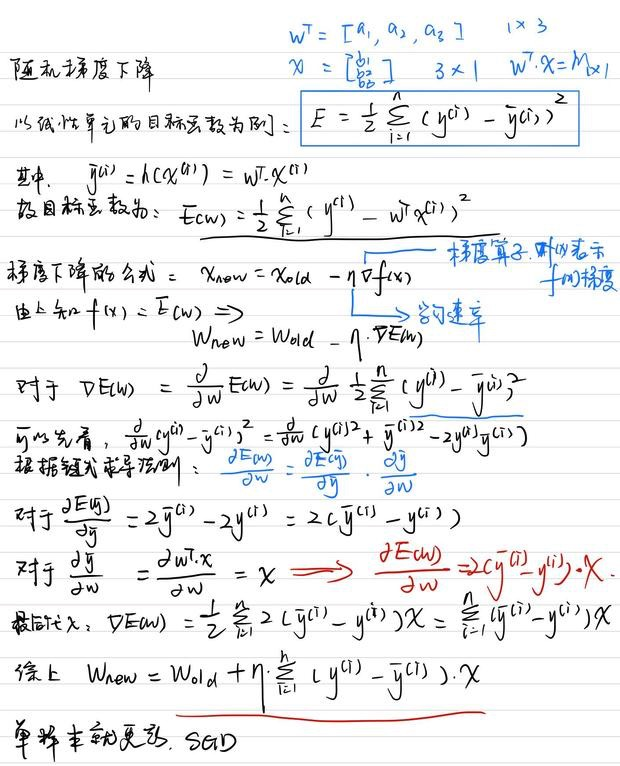## 实现数字分类模型

  1 2 3 4 5 6 7 8 9 10 11 12 13 14 15 16 17 18 19 20 21  #!/usr/bin/env python import random import numpy as np class Network(object): def __init__(self, sizes): """ 初始化 :param sizes: 如果想要初始化一个层数为3，里面神经元数量分别为：2,3,1 的神经网络，那么 sizes = [2，3，1] """ self.num_layers = len(sizes) self.sizes = sizes self.biases = [np.random.randn(y, 1) for y in sizes[1:]] self.weights = [np.random.randn(y, x) for x, y in zip(sizes[:-1], sizes[1:])] 

 1 2 3  def sigmoid(z): """The sigmoid function.""" return 1.0/(1.0+np.exp(-z)) 

  1 2 3 4 5 6 7 8 9 10 11 12 13 14 15 16 17 18  import mnist_loader, network training_data, validation_data, test_data = mnist_loader.load_data_wrapper() net = network.Network([784, 30, 10]) net.SGD(list(training_data), 30, 10, 3.0, test_data=list(test_data)) # Output Epoch 0: 9023 / 10000 Epoch 1: 9222 / 10000 Epoch 2: 9312 / 10000 Epoch 3: 9312 / 10000 Epoch 4: 9383 / 10000 Epoch 5: 9421 / 10000 Epoch 6: 9432 / 10000 Epoch 7: 9421 / 10000 Epoch 8: 9454 / 10000 Epoch 9: 9447 / 10000